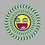# Around the clock.

The following is taken from the 2nd round of the 2014 South African Maths Olympiad:

How many times in a 24-hour day do the hands on a 12-hour clock point in exactly the same direction?Note by Victor Spirou
5 years, 4 months ago

This discussion board is a place to discuss our Daily Challenges and the math and science related to those challenges. Explanations are more than just a solution — they should explain the steps and thinking strategies that you used to obtain the solution. Comments should further the discussion of math and science.

When posting on Brilliant:

• Use the emojis to react to an explanation, whether you're congratulating a job well done , or just really confused .
• Ask specific questions about the challenge or the steps in somebody's explanation. Well-posed questions can add a lot to the discussion, but posting "I don't understand!" doesn't help anyone.
• Try to contribute something new to the discussion, whether it is an extension, generalization or other idea related to the challenge.

MarkdownAppears as
*italics* or _italics_ italics
**bold** or __bold__ bold
- bulleted- list
• bulleted
• list
1. numbered2. list
1. numbered
2. list
Note: you must add a full line of space before and after lists for them to show up correctly
paragraph 1paragraph 2

paragraph 1

paragraph 2

[example link](https://brilliant.org)example link
> This is a quote
This is a quote
    # I indented these lines
# 4 spaces, and now they show
# up as a code block.

print "hello world"
# I indented these lines
# 4 spaces, and now they show
# up as a code block.

print "hello world"
MathAppears as
Remember to wrap math in $$ ... $$ or $ ... $ to ensure proper formatting.
2 \times 3 $2 \times 3$
2^{34} $2^{34}$
a_{i-1} $a_{i-1}$
\frac{2}{3} $\frac{2}{3}$
\sqrt{2} $\sqrt{2}$
\sum_{i=1}^3 $\sum_{i=1}^3$
\sin \theta $\sin \theta$
\boxed{123} $\boxed{123}$

Sort by:

Do they seriously give such easy question in the S.A M.O? I could solve this even 3 years back!

- 5 years, 4 months ago

2nd round 2nd question but there's also a 3rd round and a camp in the junior section

- 5 years, 4 months ago

U must take a look at India's M.O papers at AoPS.. They are far more tougher than this!

- 5 years, 4 months ago

How many papers are written in India?

- 5 years, 4 months ago

- 5 years, 4 months ago

u can get them here

- 5 years, 4 months ago

I feel you may not know there are 20 questions in the SAMO. I saw most of yours have 6

- 5 years, 4 months ago

24

- 5 years, 4 months ago

I also thought that,but remembered the second hand.

- 5 years, 4 months ago

An intuitive answer that I haven't found justification for:

In $12$ hours, the minute hand travels $12$ times the circumference and the hour hand travels $1$ time, so the minute hand will cross the hour hand $11$ times, and those crossings are exactly when the two hands point in the same direction. Just multiply this by $2$ since there are $24$ hours.

- 5 years, 4 months ago

Thanks for the clear explanation @josh silverman provided more than enough justification

- 5 years, 4 months ago

The angular velocity of the minute hand and hour hand are $\omega_m = 2\pi\text{ hr}^{-1}$ and $\omega_h = \frac{2\pi}{12}\text{hr}^{-1}$, respectively.

The first intersection will occur when $\omega_m t - 2\pi = \omega_h t$, i.e. when $t = \frac{2\pi}{\omega_m- \omega_h} \approx 1.09\text{ hr}$.

As soon as an intersection happens, we have the same problem again, and the next intersection will take the same amount of time as the first. $24/1.09\approx 22.02$ which means the crossing will occur 22 times..

Staff - 5 years, 4 months ago

Why isn't it $\omega_m t - 2\pi = \omega_h t$? I mean, the LHS shows the distance traveled by the minute hand minus one circumference and the RHS shows the path traveled by the hour hand, which is speed times time.

- 5 years, 4 months ago

Typo, you are right

Staff - 5 years, 4 months ago

That means you'll have to change the expression of $t$ and then we have $t\approx 1.09 \text{hr}$ ($\frac{13}{12}$ becomes $\frac{12}{11}$) instead, but that doesn't change the answer.

- 5 years, 4 months ago

indeed, thanks for pointing that out

Staff - 5 years, 4 months ago

That's Brilliant

- 5 years, 4 months ago